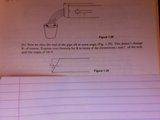# Law of sine/cosines to find resultant force

## Homework Statement

So I am using Law of sine/cosines to find resultant force R and its direction.My teacher gave me a hint to decompose the 600 and 800 into x and y components...but I have done this and cannot see what it helps me to derive? Anyone else see it?

Casey

Also, I have drawn parellogram law

## Answers and Replies

I just don't see the relationship here. It looks like the y components might add up to the y component of R...but I am not sure how to prove it or if that can even help me here.

I'm going postal as we speak....I just thought you should know.

So if A+B=R then $A_x+B_x=R_x$ and $A_y+B_y=R_y$ and $$R=\sqrt{(R_x^2+R_y^2)}$$

Is this what I just read?! If so I did this earlier and got the wrong answer...but most likly because of a stupid mistake.

Is this correct though?

When you add vectors graphically, which way must the be aligned? Tail to Tip. Otherwise, you will have a sign problem and add when you should subtract.

So,

Rx = Ax + Bx, but A (the 600 N vector) is tail to tail with the B vector, so what does this tell you?